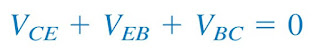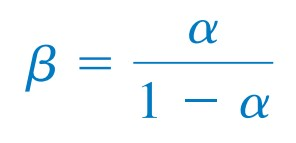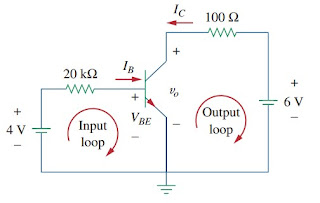omG8HI9LSKFW6lhCj8prTs0Z6lUhdOT9Jhi1Sf4m

# DC Transistor Circuits

It is very common of us dealing with electonic products on a daily basis and get some experience with personal computers. A basic component for the integrated circuits found in these electronics and computers is the active, three-terminal device known as the transistor. Understanding the transistor is essential before an engineer can start an electronic circuit design.

## Transistor

You can see in Figure.(1), some various kinds of transistors commercially available. There are two basic types of transistors : bipolar junction transistors (BJTs) and field-effect transistors (FETs).Figure 1. Transistors
This time we will cover only the BJTs, which were the first of the two and are still used today. Our objective is to learn about BJTs and able to apply the technique developed until now to analyze dc transistor circuits.
There are two types of BJTs: npn and pnp, with their circuit symbol as shown in Figure.(2). Each type has three terminals, designated as emitter (E), base (B), and collector (C). For npn transistor, the currents and voltages of the transistor are specified as in Figure.(3).Figure 3. Transistor equivalent circuit
Applying KCL to Figure.(3a) gives(1)
where IE, IC, and IB are emitter, collector, and base currents, respectively. Similarly, applying KVL to Figure.(3b) gives(2)
where VCE, VEB, and VBC are collector-emitter, emitter-base, and base-collector voltages. The BJT can operate in one of three modes: active, cutoff, and saturation.
When transistors operate in the active mode, typically VBE = 0.7 V,(3)
where α is called the common-base current gain. In Figure.(3), α denotes the fraction of electrons injected by the emitter that are collected by the collector. Also,(4)
where β is known as the common-emitter current gain. The α and β are characteristic properties of a given transistor and assume constant values for that transistor. Typically, α takes values in the range of 0.98 to 0.999, while β takes value in the range of 50 to 1000. From Equations.(1) to (4), it is evident that(6)
These equation show that, in the active mode, the BJT can be modeled as a dependent current-controlled current source. Thus, in circuit analysis, the dc equivalent model in Figure.(4b) may be used to replace the npn transistor in Figure.(4a).
Since β in Equation.(6) is large, a small base current controls large current in the output circuit. Consequently, the bipolar transistor can serve as an amplifier, producing both current gain and voltage gain. Such amplifiers can be used to furnish a considerable amount of power to transducers such as loudspeakers or control motors.Figure 4. Transistor equivalent circuit
It should be observed in the following examples that one cannot directly analyze transistor circuits using nodal analysis because of the potential difference between the terminals of the transistor. Only when the transistor is replaced by equivalent model can we apply nodal analysis.

## DC Transistor Example

For better understanding, let us review example below:
Find IC, IB ,and vo in the transistor circuit of Figure.(5). Assume that the transistor operates in the active mode and that β = 50.Figure 5
Solution :
For the input loop, KVL gives
Since VBE = 0.7 V in the active mode,
But
For the output loop, KVL gives
Note that vo = VCE in this case.

Untuk Bahasa Indonesia baca Transistor : Fungsi Transistor dan Cara Mengukur Transistor.
Related Posts
SHARE